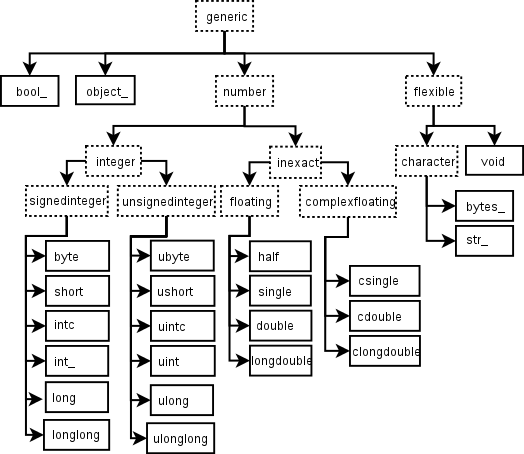# # The Scalar in NumPy

In NumPy, a scalar is any object that you put in an array. It's similar to the concept in linear algebra, an element of a field which is used to define a vector space. NumPy ensures all scalars in an array have same types. It's impossible one scalar having type int32, the other scalars having type int64. In other words, NumPy is homogeneous.

A NumPy scalar object is an instance of `np.generic`, or whose type is in `np.ScalarType`.

``````def isscalar(num):
if isinstance(num, generic):
return True
else:
return type(num) in ScalarType
``````

We can test if an object is a scalar by `isscalar()` function.

``````>>> import numpy as np
>>> np.isscalar(np.int64(1.0))
True
>>> np.isscalar(1)
True
>>> int in np.ScalarType
True
>>> np.ScalarType
(<class 'int'>, <class 'float'>, <class 'complex'>, <class 'int'>, <class 'bool'>, <class 'bytes'>, <class 'str'>, <class 'memoryview'>, <class 'numpy.complex64'>, ...
``````

There is a hierarchy of NumPy built-in scalar types. It gives us the power of testing a more generic type of a scalar. For example, the two scalar below are all of `np.number` type.

``````>>> isinstance(np.float_(1), np.number)
True
>>> isinstance(np.int_(1), np.number)
True
``````The names in this hierarchy are aliases to their actual C pointers on a particular platform. For example, on my laptop, `int_` is an alias to `int64`; `intc` is an alias to `int32`. It might varies on your platform. The numbers in the scalar type names indicates the bit-width in memory.

Interestingly, the array scalars can have some array-like attributes. The only missing attribute is `ctype`.

``````>>> np.int64(1).ndim # the number of dimensions
0
>>> np.int64(1).data # the data buffer
<memory at 0x104ec6100>
>>> np.int64(1).size # the number of elements in gentype
1
>>> np.int64(1).itemsize # the length of elements in bytes
8
>>> np.int64(1).shape # tuple of array dimensions
()
>>> np.int64(1).strides # tuple of bytes steps in each dimensions
()
>>> np.int64(1).flags
C_CONTIGUOUS : True
F_CONTIGUOUS : True
OWNDATA : True
WRITEABLE : False
ALIGNED : True
WRITEBACKIFCOPY : False
UPDATEIFCOPY : False
``````

Similarly, array scalars can be indexed and have the same methods as arrays, except that they're not settable. Internally, NumPy convert the scalar as if it's a zero dimensional array first, and then apply the methods.